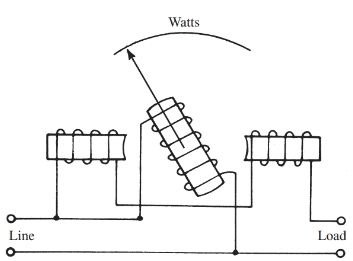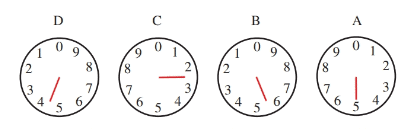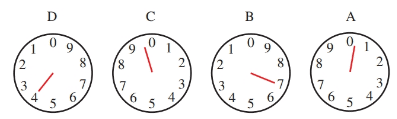# Electrodynamometer Type Wattmeter Working Principle

Want create site? Find Free WordPress Themes and plugins.

Power in an electric circuit is equal to the product of the voltage and the current. To devise a meter that measures watts, a movement similar to the D’Arsonval movement can be used. The permanent magnetic field found in the D’Arsonval movement, however, is replaced with coils from an electromagnet. This type of meter is referred to as an electrodynamometer movement.

A circuit diagram of a simple electrodynamometer movement is shown in Figure 1. This arrangement creates a wattmeter, a meter that measures the instantaneous power. A moving coil, with the proper multiplier resistance, is connected across the voltage in the circuit.

The coils of the electromagnet are connected in series with the circuit under measurement. The action between the two magnetic fields is proportional to the product of the voltage and the current. Deflection of the indicating needle is read on a calibrated scale in watts.Figure 1. Diagram of a wattmeter.

While wattmeter measures the instantaneous power used in a circuit, a watt-hour meter measures the amount of power used in a given time. It is installed by a power company on the outside of a home or business. Since a watt-hour is a very small unit, standard utility meters read in kilowatt-hours (kWh), or 1000 watt-hours.

$\text{number of kW=}\frac{E\times I}{1000}$

Electric power consumed is purchased at current rates per kWh.

The watt-hour meter is a complicated type of induction motor. It uses field coils in series with the line current and also field coils connected across the line voltage. An aluminum disk rotates within these fields at a rate proportional to the power consumed. The disk is geared to an indicating dial. The dial shows the amount of power used.

To read the watt-hour meter, see Figure 2. Dial A, on the right, reads from 0–10 units of kilowatt hours. For each revolution of dial A, dial B advances one. For each revolution of dial B, dial C advances one. For each revolution of dial C, dial D advances one. Mathematically, dial A represents units of one; dial B represents units of ten; dial C represents units of one hundred; dial D represents units of one thousand.Figure 2. The kilowatt hours indicated equal 4255. When the dial indicator rests between numbers, the lower number is selected.

Be careful when reading the meter. Note that two of the dials read clockwise (A and C), and two of the dials read counterclockwise (B and D). This arrangement is because the gears for the dials are connected directly to each other.

Read the meter illustrated in Figure 2:

Dial A points to 5.

Dial B points between 5 and 6.

Dial C points between 2 and 3.

Dial D points between 4 and 5.

Therefore, the correct reading would be 4255 kWh. Notice that when the indicating arrow is between numbers, the lower number should be used.

At times it is difficult to determine if the arrow has reached the number to which it is pointing or if the arrow has just approached the number. To make your determination, look to the dial immediately to the right of the dial in question. If the arrow on the dial to the right is between 9 and 0, then the number is still the lower value. If the arrow is past the 0, then the number is the larger value.

Use Figure 3 as an example. The arrow in dial D appears to be pointing to the number 4. To determine if it is a 4 value or still a 3, look at the dial immediately to its right, dial C. Dial C has not quite reached 0. It is still between the 9 and the 0. Thus, dial D is actually still a 3.

To practice reading meters, why not read the meter at your home each day for several days and compute the power used?

The power consumed is found by subtracting the previous reading from the present reading. The difference between the two readings is the number of kilowatt- hours of power used over the length of time between your two readings.Figure 3. This watt-hour meter reads 3970 kWh. To determine if dial D is really a four, you must look at dial C. Since dial C is between the nine and the zero, dial D is still three. It will become a four when the dial C indicator passes zero and rests between the zero and one. Dial B indicates seven. Dial A verifies that dial B is a seven and not still a six because dial A indicator is at rest between the zero and one.

Did you find apk for android? You can find new Free Android Games and apps.